# Line Graphs

GCSEKS3Level 4-5AQAEdexcelOCRWJEC

## Line Graphs

A line graph is used to compare two sets of data that are related in some way. Most commonly, a line graph is used to show how something changes over time – maybe over the course of a week, a year, or even longer.

Having an understanding of scatter graphs will help with this topic.

Level 4-5GCSEKS3AQAEdexcelOCRWJEC

## Drawing a Line Graph

Drawing a line graph is fairly simple and builds on our previous knowledge of scatter graphs.

Example:

David collected data on how much sleep he got each night for a week. His results are collected in the table shown below. Construct a line graph to represent his data.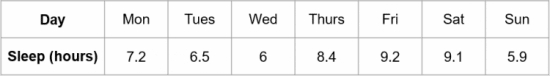When constructing a line graph, we typically choose to put the independent variable (i.e. the thing that we control) on the $x$-axis, whilst the dependent variable (the thing we’re measuring) goes on the $y$-axis. So, our line graph will have

• The days of the week on the $x$-axis, equally spaced
• The number of hours of sleep on the $y$-axis
• Axes labels
• A title

Then, to make this into a line graph, we need to connect each of the individual points using straight lines. The result should look like the graph below.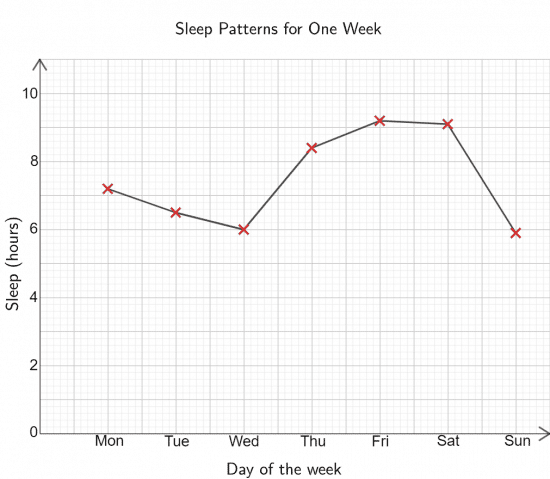Level 4-5GCSEKS3AQAEdexcelOCRWJEC

## Line Graphs Example Questions

Our line graph should have the months on the $x$-axis and the temperature on the $y$-axis. It should also have the axes clearly labelled and an appropriate title at the top (don’t worry if yours is slightly different from my choice).

With all points plotted correctly and joined with straight lines, the line graph should look like: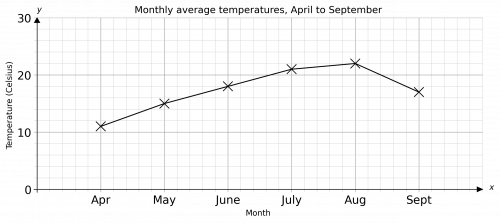Gold Standard Education

The first mistake Roger made is that he didn’t label one of his axes.

The second mistake he made is that he plotted the $2014$ point at $600$ when it should be at $700$.Gold Standard Education

The line graph should have the days on the $x$-axis and the Number of TVs sold on the $y$-axis. It should also have the axes clearly labelled and an appropriate title at the top.

With all points plotted correctly and joined with straight lines, the line graph should look like: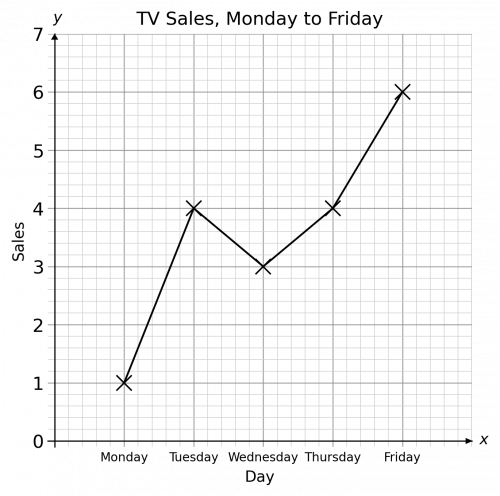Gold Standard Education

The line graph should have the year on the $x$-axis and the population on the $y$-axis. It should also have the axes clearly labelled and an appropriate title at the top.

With all points plotted correctly and joined with straight lines, the line graph should look like: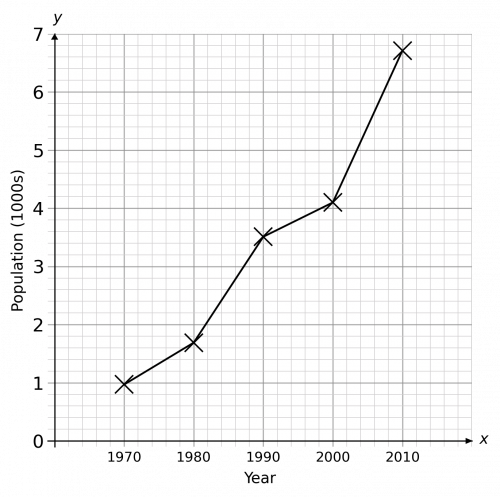Gold Standard Education

The line graph should have the month on the $x$-axis and the rainfall on the $y$-axis. It should also have the axes clearly labelled and an appropriate title at the top.

With all points plotted correctly and joined with straight lines, the line graph should look like: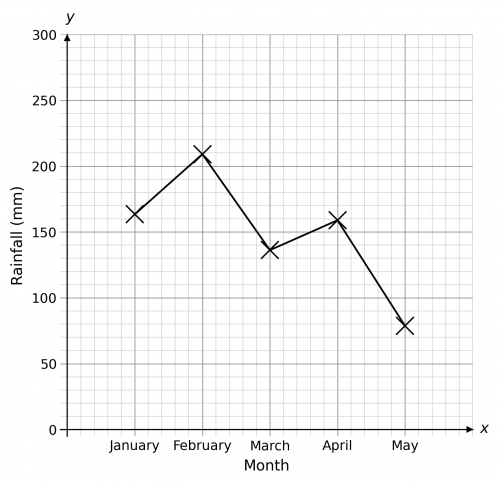Gold Standard Education

## Line Graphs Worksheet and Example Questions

### (NEW) Line Graphs Exam Style Questions - MME

Level 4-5GCSENewOfficial MMEProduct